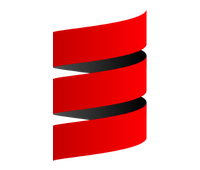in the wake of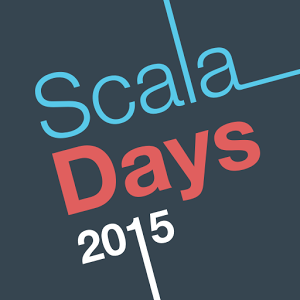MAIN THEMES

• Reactive Applications
• Big Data/Fast Data
• FP Scala
• Scala in practice
• Distributed Systems

where's scala going

highlights from Odersky's keynote

• Beginning of the reactive platform
• Scala-specific platform with TASTY
(Serialized Typed Abstract Syntax Trees)
• DOTC compiler (close to completion)
• Type unions (U&T and U|T)
• Implicits that compose
(implicit function types)

• Moving towards purity (in FP sense)

A lot of awesome slides @ http://www.slideshare.net/Odersky

SCALA platform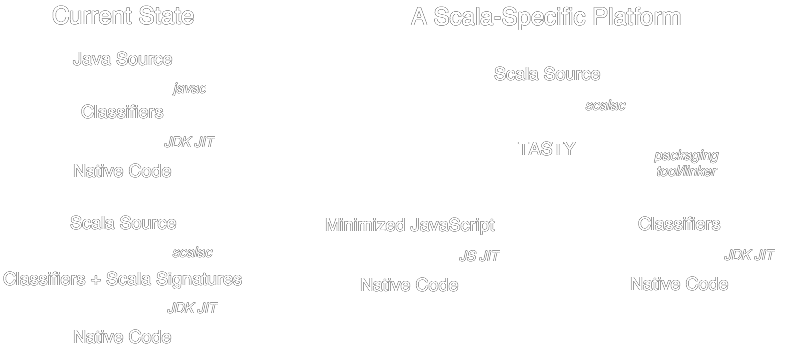TASTY & DOTC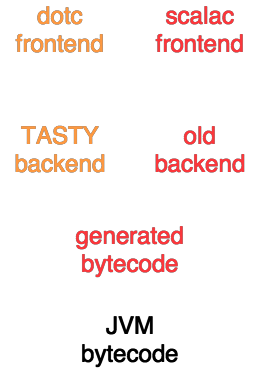Dependent Object Types calculus paper

life beyond the illusion of present

highlights from Jonas Boner's keynote

• philosophical talk about notion of time and consistency in distributed systems
• CR UD
• Vector Clocks
• CALM: consistency as logical monotonicity
• CRDT: cummutative replicated data types

CALM

In general terms, the CALM principle says that:

• logically monotonic distributed code is  eventually consistent without any need for coordination protocols (distributed locks, two-phase commit, paxos, etc.)
• eventual consistency can be  guaranteed in any program by protecting non-monotonic statements (“points of order”) with coordination protocols.

Lambda Architecture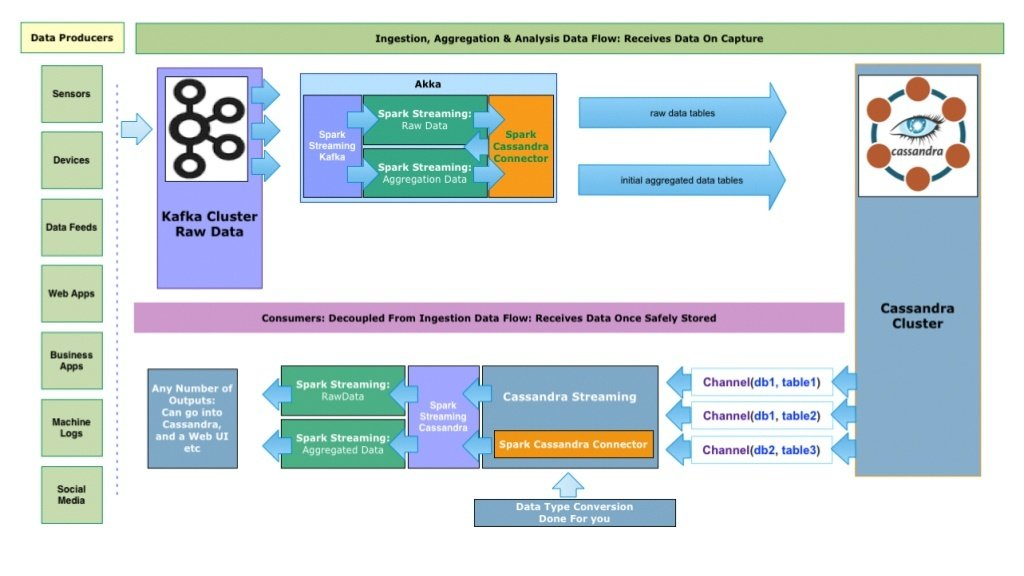def f1: Future[Option[Int]] = ???
def f2: Future[Option[Int]] = ???
def f3: Future[Option[Int]] = ???

val result = for{
a <- OptionT(f1)
b <- OptionT(f2)
c <- OptionT(f3)
} yield a + b + c

val finOption: Future[Option[Int]] = result.run

def f1: Future[String \/ Int] = ???
def f2: Option[Int] = ???
def f3: Future[Int] = ???

val result = for{
a <- EitherT(f1)
b <- EitherT(Future(f2 \/> "B is missing"))
c <- EitherT(f3.map(v => \/.right(v))
} yield a + b + c

val finEither: Future[String \/ Int] = result.run

case class FutureOption[A](contents: Future[Option[A]]){

def flatMap[B](fn: A => FutureOption[B]) = FutureOption{
contents.flatMap{
case Some(value) => fn(value).contents
case None => Future.successful(None)
}
}

def map[B](fn: A => B) = FutureOption{
contents.map{ option =>
option.map(fn)
}
}
}

Monad is a typeclass with methods:
• map
• flatMap
• create

Great slides on this topic here

understanding the backend of big data

cluster resource management

• Scala (and FP in general) is a perfect fit for
distributed computing
• cluster resources are used for about 5-10% ,
• DCOS
dcos package install spark

dcos package install hdfs
check out mesosphere.com for more details

essential scala

Six Core Concepts for Learning Scala by Noel Welsh

1. Expressions, types, & values
2. Objects and classes
3. Algebraic data types
4. Structural recursion
5. Sequencing computation
6. Type classes

Slides available here

algebraic data types

• Goal: translate data description into code
• Model data with logical ORs and ANDs
• Two patterns: product types(ANDs) and sum types(ORs)
• Sum and product together make algebraic data types

//product type: A has a B and C
final case class A(b: B, c: C)

//sum type: A is a B or C
sealed trait A
final case class B() extends A
final case class C() extends A

//Example: a Calculation either succesfull and has value or failed and has an errorsealed trait Calculationfinal case class Success(value: Int) extends Calculationfinal case class Failure(msg: String) extends Calculation

structural recursion

• Goal: transform algebraic data type
• Two patterns:
pattern-matching & polymorphism

//pattern matching

sealed trait A {
def doSomething: H = {
this match {
case B(d, e) => doB(d, e)
case C(f, g) => doC(f, g)
}
}
}
final case class B(d: D, e: E) extends A
final case class C(f: F, g: G) extends A

STRUCTURAL RECURSION cont'd

//polymorphism
sealed trait A {
def doSomething: H
}
final case class B(d: D, e: E) extends A {
def doSomething: H =
doB(d, e)
}
final case class C(f: F, g: G) extends A {
def doSomething: H =
doC(f, g)
}

structural recursion example

sealed trait Calculation {
this match {
case Success(v) => Success(v + value)
case Failure(msg) => Failure(msg)
}
}

final case class Success(value: Int) extends Calculation
final case class Failure(msg: String) extends Calculation

Summary:

• Processing algebraic data types immediately follows from the structure of the data
• Can choose between pattern matching and polymorphism
• Pattern matching (within the base trait) is usually preferred

sequencing computations

• Goal: patterns for sequencing computations
• Functional programming is about transforming values
• ... without introducing side-effects
• A => B => C
• Three patterns: fold, map, and flatMap
• Fold is abstraction over structural recursion

DIY fold example

sealed trait A {
def doSomething: H = {
this match {
case B(d, e) => doB(d, e)
case C(f, g) => doC(f, g)
}
}
}

final case class B(d: D, e: E) extends A
final case class C(f: F, g: G) extends A
sealed trait A {
def fold(doB: (D, E) => H, doC: (F, G) => H): H = {
this match {
case B(d, e) => doB(d, e)
case C(f, g) => doC(f, g)
}
}
}

final case class B(d: D, e: E) extends A
final case class C(f: F, g: G) extends A

DIY fold example II

sealed trait Result[A] {
def fold[B](s: A => B, f: B): B =
this match {
Success(v) => s(v)
Failure() => f
}
}

final case class Success[A](value: A) extends Result[A]
final case class Failure[A]() extends Result[A]

• fold is a generic transform for any algebraic data type
• but it's not always the best choice
• not all data is algebraic data type
• there're another methods easier to use (map and flatMap)

essential scala conclusions

• Scala  is simple
• 3 patterns are 90% of code
• 4 patterns are 99% of code

a purely functional approach to building large applications

by Noel Markham

(good post here)
• Kleisli arrow
• "wiring" functions together
• scalaz, shapeless, scalacheck

Code and presentation available here

Easy Scalable Akka applications

• CQRS & ES (event sourcing)
• Akka persistence over Cassandra
• Akka clustering
• Gatling stress tool
template akka-dddd-template

Awesome approach to replace CRUD with storing WAL and snapshots to represent domain entities state and persist it in Cassandra

Slides available here

some other awesome topics

• Spores
• Project Gålbma: Actors vs. Types
• Finagle in SoundCloud
• Why Spark Is the Next Top (Compute) Model
• Scala.js
• The Reactive Streams Implementation Landscape

Videos and slides avalable @ Parleys

Some other links to slides @ cakesolutions blog

wraping up

• Scala language is now stable in terms of API changes
and moving towards being more functional as well
as growing a platform like JEE [in good sense =) ]
• Scala is used as implementation language in
major and emerging distributed data
processing/computing frameworks
• Scalaz is used in wide variety of projects for
proper abstractions or just for making code
more concise and reusable (\/, transformers)
• There're a lot of Scala libraries/frameworks
forming mature ecosystem (e.g. Akka and Play)
which is used for building large applications

be well and code in scala